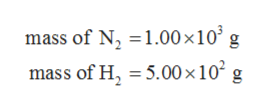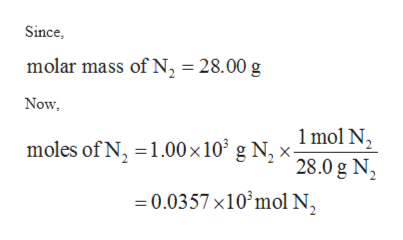# Ammonia is produced from the reaction of nitrogen and hydrogen according to the following equation:N2  +  3H2 --> 2NH3b.What mass of the excess reagent would remain unreacted from a mixture of 1.00 x 103g N2 and 5.00 x 102g H2?

Question
3 views

Ammonia is produced from the reaction of nitrogen and hydrogen according to the following equation:

N2  +  3H2 --> 2NH3

b.What mass of the excess reagent would remain unreacted from a mixture of 1.00 x 103g N2 and 5.00 x 102g H2

check_circle

Step 1

The values are given as follows:help_outlineImage Transcriptionclosemass of N 1.00 x 10 g mass of H 5.00 x 10? g fullscreen
Step 2

The balanced chemical equation is given as follows:

Step 3

Initially, the number of moles of N2 can...help_outlineImage TranscriptioncloseSince molar mass of N, 28.00 Now 1mol N moles of N, 1.00 x 10 gN* 28.0 g N 0.0357 x10'mol N fullscreen

### Want to see the full answer?

See Solution

#### Want to see this answer and more?

Solutions are written by subject experts who are available 24/7. Questions are typically answered within 1 hour.*

See Solution
*Response times may vary by subject and question.
Tagged in
ScienceChemistry

### General Chemistry